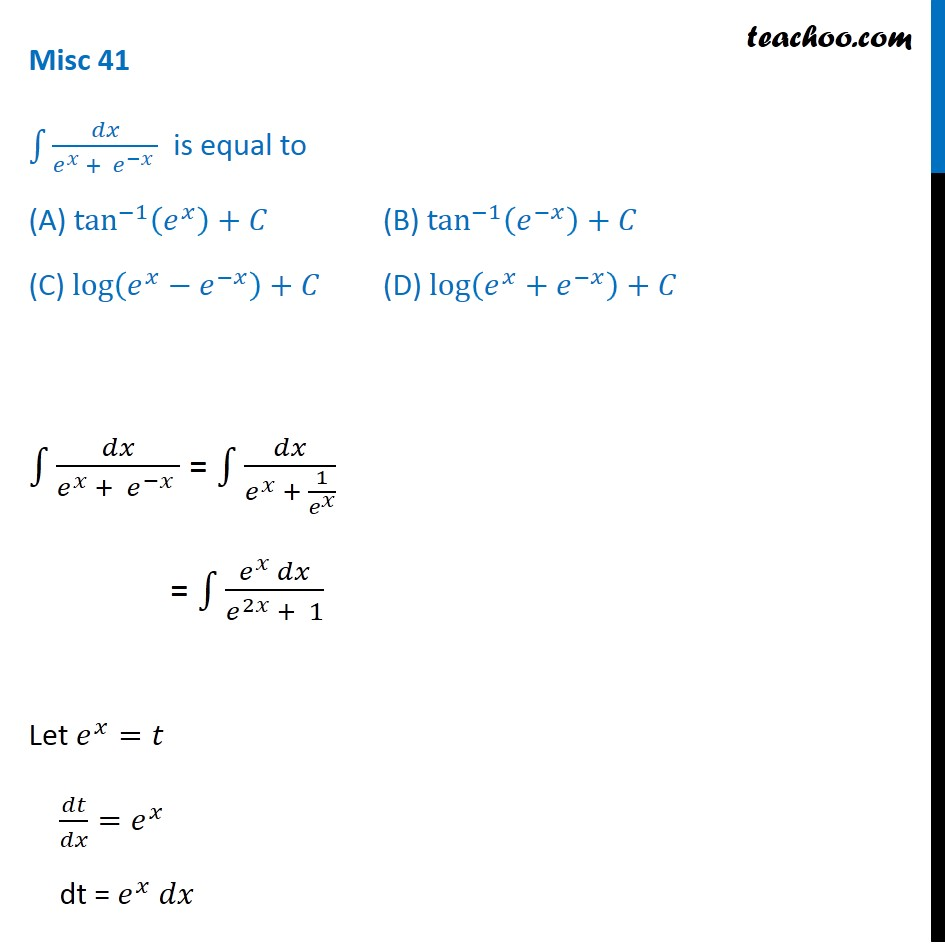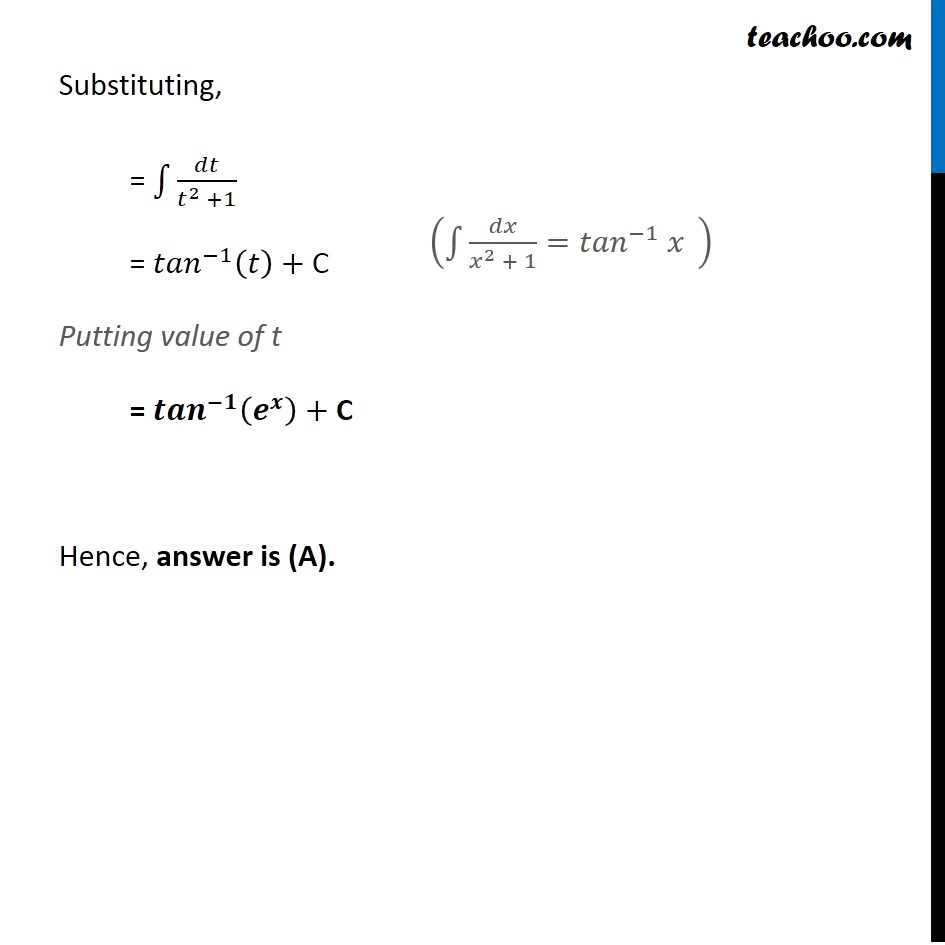Miscellaneous

Chapter 7 Class 12 Integrals
Serial order wiseLearn in your speed, with individual attention - Teachoo Maths 1-on-1 Class

### Transcript

Misc 41 ∫▒𝑑𝑥/(𝑒^𝑥 + 𝑒^(−𝑥) ) is equal to (A) tan^(−1) (𝑒^𝑥 )+𝐶 (B) tan^(−1)⁡〖(𝑒^(−𝑥) )+𝐶〗 (C) log⁡(𝑒^𝑥−𝑒^(−𝑥) )+𝐶 (D) log⁡(𝑒^𝑥+𝑒^(−𝑥) )+𝐶 ∫▒𝑑𝑥/(𝑒^𝑥 + 𝑒^(−𝑥) ) = ∫▒𝑑𝑥/(𝑒^𝑥 + 1/𝑒^𝑥 ) = ∫1▒(𝑒^𝑥 𝑑𝑥)/(𝑒^2𝑥 + 1) Let 𝑒^𝑥=𝑡 𝑑𝑡/𝑑𝑥=𝑒^𝑥 dt = 𝑒^𝑥 𝑑𝑥 Substituting, = ∫1▒𝑑𝑡/(𝑡^2 +1) = 〖𝑡𝑎𝑛〗^(−1) (𝑡)+ C Putting value of t = 〖𝒕𝒂𝒏〗^(−𝟏) (𝒆^𝒙 )+ C Hence, answer is (A). (∫1▒〖𝑑𝑥/(𝑥^2 + 1)=〖𝑡𝑎𝑛〗^(−1) 𝑥〗 " " )